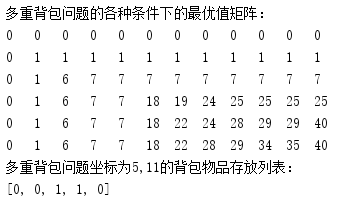背包问题

$n$$n$种重量和价值分别为$weigh{t}_{i}$$weight_i$$valu{e}_{i}$$value_i$的物品，假设有一个容量为$w$$w$的背包，求怎么样装这个背包，才能使得$\sum _{1}^{n}{k}_{i}\ast weigh{t}_{i}（其中{k}_{i}为0到物品的数量至多有多少）$$\sum_1^n{k_i*weight_i}（其中k_i为0到物品的数量至多有多少）$不超过背包的总重量，并获得最大的价值$\sum _{1}^{n}{k}_{i}\ast valu{e}_{i}$$\sum_1^n{k_i*value_i}$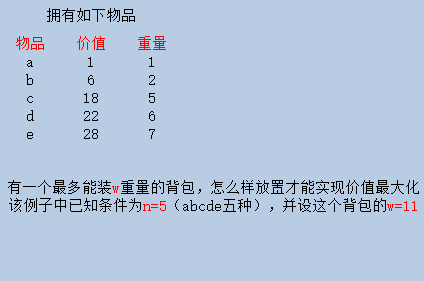1、假设这里每种物品至多只能取一次，并且我们先不管这个背包真实容量11，我们假设这个背包容量其实只有1，并且此时此刻可选物品也只有a，显然，在这种情况下，a能放入这个背包，并得到了价值1。这时候假设我们可选物品多了一件b，容量仍为1，但b的重量大于1，放不下，与此类似，我们可知，即使可选物品变成5件，因为其他物品的重量都超过了当前这个背包容量1，我们可放的物品仍然只有a，总的价值还是为1。

2、这时候我们假设背包容量变成了2，一开始还是只能放a，得到的价值为1。这时候，假设b也可选了，即此时ab均可选，那么因为b的重量小于等于2，此时价值最大的方式就是把刚刚放的a拿出来，放入b，就可以得到价值6。当$cde$$cde$也可选，因为容量超限，显然不能再有更好的放置方案。

3、接着，我们假设背包容量变成了3，起初只有a一种物品，那么能放入，得到的价值为1，这时候再来一件b，a+b仍然不超过容量3，因此可以得到价值7

$opt\left(i,w\right)=max\left(在第i件物品未出现时所能获得的最大价值，在第i件物品出现后所能获得的最大价值\right)$

$opt\left(i,w\right)=max\left\{opt\left(i-1,w\right),opt\left(i-1,w-weigh{t}_{i}\right)+valu{e}_{i}\right\}$

问题具体化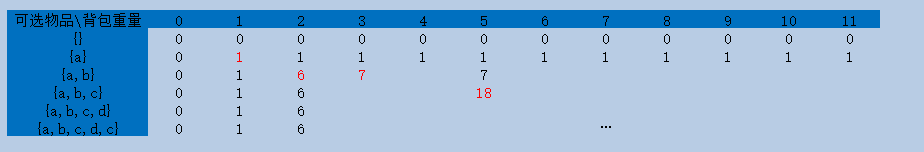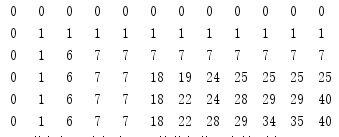01背包问题

$\left(1\right).opt\left(i,w\right)=0\phantom{\rule{2em}{0ex}}if\phantom{\rule{thickmathspace}{0ex}}i\le 0$$(1).opt(i,w)=0\qquad if \; i\leq0$
$\left(2\right).opt\left(i,w\right)=opt\left(i-1,w\right)\phantom{\rule{2em}{0ex}}if\phantom{\rule{thickmathspace}{0ex}}weigh{t}_{i}\ge w$$(2).opt(i,w)=opt(i-1,w)\qquad if \; weight_i\geq w$
$\left(3\right).opt\left(i,w\right)=max\left\{opt\left(i-1,w\right),opt\left(i-1,w-weigh{t}_{i}\right)+valu{e}_{i}\right\}\phantom{\rule{2em}{0ex}}otherwise$$(3).opt(i,w)=max\lbrace opt(i-1,w),opt(i-1,w-weight_i)+value_i \rbrace \qquad otherwise$

def main():
w = 11  # 背包容量
n = 5  # 物品种类/个数
# 对应为物品a,b,c,d,e
value = [1, 6, 18, 22, 28]  # 价值
weight = [1, 2, 5, 6, 7]  # 开销

# 01问题
zero_one_problem(w, n, weight, value)

# 01问题
def zero_one_problem(w, n, weight, value):
# 创建一个大小为w+1 * n+1 的全零矩阵，用来放结果
matix = [[0 for i in range(w + 1)] for j in range(n + 1)]

# 背包种类个数开始上升
for row in range(1, n + 1):
# 容量从1开始增长
for col in range(1, w + 1):
# 先看看新来的花费是不是大于当前容量
if (weight[row - 1] > col):
# 如果是，就在前面找最大值
matix[row][col] = matix[row - 1][col]
else:
# 如果不是，就看看新增一个物品价值牛逼点，还是不用这个物品也很厉害
matix[row][col] = max(matix[row - 1][col], value[row - 1] + matix[row - 1][col - weight[row - 1]])
print('0/1背包问题的各种条件下的最优值矩阵：')
for it in matix:
for i in it:
print(i, '\t', end='')
print()
# 看看对应某一个位置对应放了什么物品
res = [0 for heh in range(n)]
row = n  # row和col为你要查的某个元素的坐标对应的物品放置列表
col = w  # 可改
# 打印矩阵
while row - 1:
if matix[row][col] == (
matix[row - 1][col - weight[row - 1]] + value[row - 1]):
res[row - 1] = 1
col = col - weight[row - 1]
row -= 1
res = int(matix[col] / value)
print('0/1背包问题坐标为%d,%d的背包物品存放列表：' % (n, w))
print(res)
print('-' * 50)

if __name__ == '__main__':
main()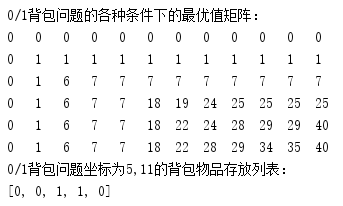完全背包问题

$\left(1\right).opt\left(i,w\right)=0\phantom{\rule{2em}{0ex}}if\phantom{\rule{thickmathspace}{0ex}}i\le 0$$(1).opt(i,w)=0\qquad if \; i\leq0$
$\left(2\right).opt\left(i,w\right)=max\left\{opt\left(i-1,w\right),opt\left(i-1,w-k×weigh{t}_{i}\right)+k×valu{e}_{i}\right\}\phantom{\rule{2em}{0ex}}otherwise$$(2).opt(i,w)=max\lbrace opt(i-1,w),opt(i-1,w-k\times weight_i)+k\times value_i \rbrace \qquad otherwise$

def main():
w = 11  # 背包容量
n = 5  # 物品种类/个数
# 对应为物品a,b,c,d,e
value = [1, 6, 18, 22, 28]  # 物品价值
weight = [1, 2, 5, 6, 7]  # 物品重量

# 完全背包问题
complete_problem(w, n, weight, value)

# 完全背包问题
def complete_problem(w, n, weight, value):
# 创建一个大小为w+1 * n+1 的全零矩阵，用来放结果
matix = [[0 for i in range(w + 1)] for j in range(n + 1)]

# 背包种类个数开始上升
for row in range(1, n + 1):
# 容量从1开始增长
for col in range(1, w + 1):
max_k = int(col / weight[row - 1])  # 不限个数，所以只要新增背包上限不超过总容量即可
for k in range(max_k + 1):
matix[row][col] = max(matix[row - 1][col],
k * value[row - 1] + matix[row - 1][col - k * weight[row - 1]])
print('完全背包问题的各种条件下的最优值矩阵：')
for it in matix:
for i in it:
print(i, '\t', end='')
print()

# 获取最优值矩阵中某个位置为行row，列col的点的物品放置列表
res = [0 for heh in range(n)]
row = n  # row和col为你要查的某个元素的坐标对应的物品放置列表
col = w  # 可改
# 打印矩阵
# 这个求解方法完全是反向思维，即上面求解倒着来，要多加理解
while row - 1:
count = int(col / weight[row - 1])
for k in range(count, 0, -1):
if matix[row][col] == (
matix[row - 1][col - weight[row - 1] * k] + k * value[row - 1]):
res[row - 1] = k
col = col - k * weight[row - 1]
break
row -= 1
res = int(matix[col] / value)
print('完全背包问题坐标为%d,%d的背包物品存放列表：' % (n, w))
print(res)
print('-' * 50)

if __name__ == '__main__':
main()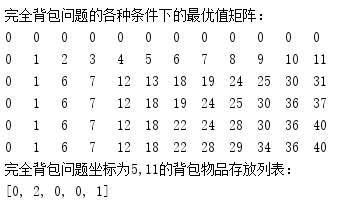多重背包问题

$\left(1\right).opt\left(i,w\right)=0\phantom{\rule{2em}{0ex}}if\phantom{\rule{thickmathspace}{0ex}}i\le 0$$(1).opt(i,w)=0\qquad if \; i\leq0$
$\left(2\right).opt\left(i,w\right)=max\left\{opt\left(i-1,w\right),opt\left(i-1,w-k×weigh{t}_{i}\right)+k×valu{e}_{i}\right\}\phantom{\rule{2em}{0ex}}otherwise$$(2).opt(i,w)=max\lbrace opt(i-1,w),opt(i-1,w-k\times weight_i)+k\times value_i \rbrace \qquad otherwise$

def main():
w = 11  # 背包容量
n = 5  # 物品种类/个数
# 对应为物品a,b,c,d,e
value = [1, 6, 18, 22, 28]  # 物品价值
weight = [1, 2, 5, 6, 7]  # 物品重量

# 多重背包问题，多了一个数量限制
num = [1, 1, 1, 1, 1]  # 背包个数，如果都是1，那么多重背包问题其实就是一个01背包问题
# num = [3, 3, 3, 3, 3]  # 背包个数，上下两个切换注释看一看
multiple_problem(w, n, weight, value, num)

# 多重背包问题
def multiple_problem(w, n, weight, value, num):
# 创建一个大小为w+1 * n+1 的全零矩阵，用来放结果
matix = [[0 for i in range(w + 1)] for j in range(n + 1)]

# 背包种类个数开始上升
for row in range(1, n + 1):
# 容量从1开始增长
for col in range(1, w + 1):
# 有限个数，所以要在不超出背包容量和限制个数的条件下选一个值
# 最小值满足木桶的短板理论，即能放多少，取决于少的那个数
max_k = min(int(col / weight[row - 1]), num[row - 1])
for k in range(max_k + 1):
matix[row][col] = max(matix[row - 1][col],
k * value[row - 1] + matix[row - 1][col - k * weight[row - 1]])
print('多重背包问题的各种条件下的最优值矩阵：')
for it in matix:
for i in it:
print(i, '\t', end='')
print()

# 获取最优值矩阵中某个位置为行row，列col的点的物品放置列表
res = [0 for heh in range(n)]
row = n  # row和col为你要查的某个元素的坐标对应的物品放置列表
col = w  # 可改
# 打印矩阵
while row - 1:
count = min(int(col / weight[row - 1]), num[row - 1])
for k in range(count, 0, -1):
if matix[row][col] == (
matix[row - 1][col - weight[row - 1] * k] + k * value[row - 1]):
res[row - 1] = k
col = col - k * weight[row - 1]
break
row -= 1
res = int(matix[col] / value)
print('多重背包问题坐标为%d,%d的背包物品存放列表：' % (n, w))
print(res)

if __name__ == '__main__':
main()